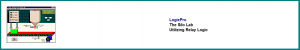# 2.3: Hands-on Activity

$$\newcommand{\vecs}{\overset { \rightharpoonup} {\mathbf{#1}} }$$ $$\newcommand{\vecd}{\overset{-\!-\!\rightharpoonup}{\vphantom{a}\smash {#1}}}$$$$\newcommand{\id}{\mathrm{id}}$$ $$\newcommand{\Span}{\mathrm{span}}$$ $$\newcommand{\kernel}{\mathrm{null}\,}$$ $$\newcommand{\range}{\mathrm{range}\,}$$ $$\newcommand{\RealPart}{\mathrm{Re}}$$ $$\newcommand{\ImaginaryPart}{\mathrm{Im}}$$ $$\newcommand{\Argument}{\mathrm{Arg}}$$ $$\newcommand{\norm}{\| #1 \|}$$ $$\newcommand{\inner}{\langle #1, #2 \rangle}$$ $$\newcommand{\Span}{\mathrm{span}}$$ $$\newcommand{\id}{\mathrm{id}}$$ $$\newcommand{\Span}{\mathrm{span}}$$ $$\newcommand{\kernel}{\mathrm{null}\,}$$ $$\newcommand{\range}{\mathrm{range}\,}$$ $$\newcommand{\RealPart}{\mathrm{Re}}$$ $$\newcommand{\ImaginaryPart}{\mathrm{Im}}$$ $$\newcommand{\Argument}{\mathrm{Arg}}$$ $$\newcommand{\norm}{\| #1 \|}$$ $$\newcommand{\inner}{\langle #1, #2 \rangle}$$ $$\newcommand{\Span}{\mathrm{span}}$$$$\newcommand{\AA}{\unicode[.8,0]{x212B}}$$

##Overview

Students download a 15-day free trial of PLC Ladder (located at PLCtrainer.net or LogicsPro). They install the software and explore its options to understand how PLC works; packet capture – protocol and transit across the network; and how to program the PLC.

### Hands-on Activity Objectives

• Describe the purpose and use of SCADA, DCS, and PCS systems.
• Describe the configuration and use of field devices used to measure critical infrastructure processes, such as flow rate, pressure, temperature, level, density, etc.
• Provide examples of HMI screens and displays used within SCADA systems.
• Describe the use and application of PLCs in automation.

## Lab Assignment

### Use PLC Simulator to explore relay logic

1. Install the LogixPro 500 PLC simulator on a Windows VM. It can be used with a 15-day free trial (available from The Learning Pit).
2. Launch the LogixPro simulator.
3. Click on the “Help” drop-down menu and select “Student Exercises.” The following web page will open.4. Under the “Student RSLogix Programming Exercises” section, select the “Relay Logic …. Introductory Exercise” option. The following web page will open.5. Complete the “LogixPro Relay Logic Introductory Lab” exercise following these instructions. You can use the printed handout instead if preferred.
6. Under the “Student RSLogix Programming Exercises” section, select the “Door Simulation …. Applying Relay Logic” option. The following web page will open.7. Complete the “LogixPro Door Simulation Lab” exercise following these instructions. You can use the printed handout instead if preferred.
8. Under the “Student RSLogix Programming Exercises” section, select the “Silo Simulator …. Applying Relay Logic to a Process” option. The following web page will open.9. Complete the “LogixPro Silo Lab” exercise following these instructions. You can use the printed handout instead if preferred.